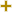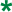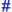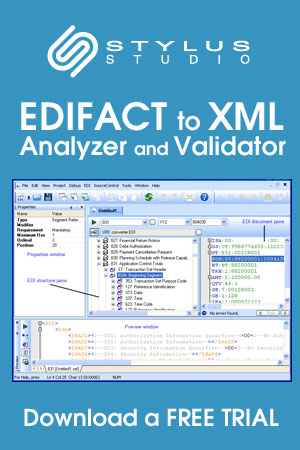# Date or time or period format codeUN/CEFACT Revision 2004B Code ListRepr:an..3
Desc:Code specifying the representation of a date, time or period.
This table also occurs in the following versions of this standard:
D00A, D00B, D01A, D01B, D01C, D02A, D02B, D03A, D03B, D04A, D04B, D93A, D94A, D94B, D95A, D95B, D96A, D96B, D97A, D97B, D98A, D98B, D99A, D99B

 2 DDMMYY Calendar date: D = Day; M = Month; Y = Year. 3 MMDDYY Calendar date: M = Month; D = Day; Y = Year. 4 DDMMCCYY Calendar date C=Century; Y=Year; M=Month; D=Day. 5 DDMMCCYYHHMM Calendar date and time: C=Century; Y=Year; M=Month; D=Day; H=Hour; M=Minute. 6 CCYYMMB Half-month: CC=century YY=year MM=month, B=1:first half month, B=2:second half month. 7 CCYYMMW Week within a calendar month: CC=century YY=year MM=month. W=1-5 first week to fifth week in a month. 8 CCYYMMDDS Shift within a calendar day: CC=century YY=year MM=month DD=day S=1-9 shift in a day. 9 CCYYMMDDPP Time period within a calendar day: CC=century YY=year MM=month DD=day PP=00-99 time period. 10 CCYYMMDDTHHMM Calendar date including time with minutes: C=Century; Y=Year; M=Month; D=Day; T=Time designator; H=Hour; M=Minutes. The character [T] shall be used as time designator to indicate the start of the representation of the time. For example: 20010912T1433. 101 YYMMDD Calendar date: Y = Year; M = Month; D = Day. 102 CCYYMMDD Calendar date: C = Century ; Y = Year ; M = Month ; D = Day. 103 YYWWD Calendar week day: Y = Year ; W = Week ; D = Day Week number 01 is always first week of January Day number 1 is always Monday. 105 YYDDD Calendar day: Y = Year ; D = Day January the first = Day 001 Always start numbering the days of the year from January 1st through December 31st. 106 MMDD Day of a month: M = Month; D = Day. 107 DDD Day's number within a specific year: D = Day. 108 WW Week's number within a specific year: W = Week. 109 MM Month's number within a specific year: M = Month. 110 DD Day's number within is a specific month: D = Day. 201 YYMMDDHHMM Calendar date including time without seconds: Y = Year; M = Month; D = Day; H = Hour; M = Minute. 202 YYMMDDHHMMSS Calendar date including time with seconds: Y = Year; M = Month; D = Day; H = Hour; m = Minutes = Seconds. 203 CCYYMMDDHHMM Calendar date including time with minutes: C=Century; Y=Year; M=Month; D=Day; H=Hour; M=Minutes. 204 CCYYMMDDHHMMSS Calendar date including time with seconds: C=Century;Y=Year; M=Month;D=Day;H=Hour;M=Minute;S=Second. 205 CCYYMMDDHHMMZHHMM Calendar date including time and time zone expressed in hours and minutes. ZHHMM = time zone given as offset from Coordinated Universal Time (UTC). 301 YYMMDDHHMMZZZ See 201 + Z = Time zone. 302 YYMMDDHHMMSSZZZ See 202 + Z = Time zone. 303 CCYYMMDDHHMMZZZ See 203 plus Z=Time zone. 304 CCYYMMDDHHMMSSZZZ See 204 plus Z=Time zone. 305 MMDDHHMM Month, day, hours, minutes; M = Month; D = Day; H = Hour; M = Minute. 306 DDHHMM Day, hours, minutes; D = Day; H = Hour; M = Minute. 401 HHMM Time without seconds: H = Hour; m = Minute. 402 HHMMSS Time with seconds: H = Hour; m = Minute; s = Seconds. 404 HHMMSSZZZ See 402 plus Z=Time zone. 405 MMMMSS Time without hours: m=minutes, s=seconds. 406 ZHHMM Offset from Coordinated Universal Time (UTC) where Z is plus (+) or minus (-). 501 HHMMHHMM Time span without seconds: H = Hour; m = Minute;. 502 HHMMSS-HHMMSS A period of time specified by giving the start time followed by the end time (both expressed by hours minutes and seconds). Data is to be transmitted as consecutive characters without hyphen. 503 HHMMSSZZZ-HHMMSSZZZ A period of time specified by giving the start time followed by the end time (both expressed by hours minutes, seconds and time zone). Data is to be transmitted as consecutive characters without hyphen. 600 CC Century. 601 YY Calendar year: Y = Year. 602 CCYY Calendar year including century: C = Century; Y = Year. 603 YYS Semester in a calendar year: Y = Year; S = Semester. 604 CCYYS Semester in a calendar year: C = Century; Y = Year; S = Semester. 608 CCYYQ Quarter in a calendar year: C = Century; Y = Year; Q = Quarter. 609 YYMM Month within a calendar year: Y = Year; M = Month. 610 CCYYMM Month within a calendar year: CC = Century; Y = Year; M = Month. 613 YYMMA To specifiy a ten-day period within a month of a year (A = ten day period). 614 CCYYMMA To specifiy a ten-day period within a month of a year, including century (A = ten day period). 615 YYWW Week within a calendar year: Y = Year; W = Week 1st week of January = week 01. 616 CCYYWW Week within a calendar year: CC = Century; Y = Year; W = Week (1st week of January = week 01). 701 YY-YY A period of time specified by giving the start year followed by the end year (both without century). Data is to be transmitted as consecutive characters without hyphen. 702 CCYY-CCYY A period of time specified by giving the start year followed by the end year (both including century). Data is to be transmitted as consecutive characters without hyphen. 703 YYS-YYS A period of time specified by giving the start semester of a year followed by the end semester of a year (both not including century). Data is to be transmitted as consecutive characters without hyphen. 704 CCYYS-CCYYS A period of time specified by giving the start semester of a year followed by the end semester of a year (both including century). Data is to be transmitted as consecutive characters without hyphen. 705 YYPYYP Format of period to be given without hyphen (P = period of 4 months). 706 CCYYP-CCYYP Format of period to be given without hyphen (P = period of 4 months). 707 YYQ-YYQ A period of time specified by giving the start quarter of a year followed by the end quarter of year (both not including century). Data is to be transmitted as consecutive characters without hyphen. 708 CCYYQ-CCYYQ A period of time specified by giving the start quarter of a year followed by the end quarter of year (both including century). Data is to be transmitted as consecutive characters without hyphen. 709 YYMM-YYMM A period of time specified by giving the start month of a year followed by the end month of a year (both not including century). Data is to be transmitted as consecutive characters without hyphen. 710 CCYYMM-CCYYMM A period of time specified by giving the start month of a year followed by the end month of a year (both including century). Data is to be transmitted as consecutive characters without hyphen. 713 YYMMDDHHMM-YYMMDDHHMM A period of time specified by giving the start time followed by the end time (format year, month, day, hour and minute). Data is to be transmitted as consecutive characters without hyphen. 715 YYWW-YYWW A period of time specified by giving the start week of a year followed by the end week of year (both not including century). Data is to be transmitted as consecutive characters without hyphen. 716 CCYYWW-CCYYWW A period of time specified by giving the start week of a year followed by the end week of year (both including century). Data is to be transmitted as consecutive characters without hyphen. 717 YYMMDD-YYMMDD A period of time specified by giving the start date followed by the end date (both not including century). Data is to be transmitted as consecutive characters without hyphen. 718 CCYYMMDD-CCYYMMDD A period of time specified by giving the start date followed by the end date (both including century). Data is to be transmitted as consecutive characters without hyphen. 719 CCYYMMDDHHMM-CCYYMMDDHHMM A period of time which includes the century, year, month, day, hour and minute. Format of period to be given in actual message without hyphen. 720 DHHMM-DHHMM Format of period to be given without hyphen (D=day of the week, 1=Monday; 2=Tuesday; ... 7=Sunday). 801 Year To indicate a quantity of years. 802 Month To indicate a quantity of months. 803 Week To indicate a quantity of weeks. 804 Day To indicate a quantity of days. 805 Hour To indicate a quantity of hours. 806 Minute To indicate a quantity of minutes. 807 Second To indicate a quantity of seconds. 808 Semester To indicate a quantity of semesters (six months). 809 Four months period To indicate a quantity of four months periods. 810 Trimester To indicate a quantity of trimesters (three months). 811 Half month To indicate a quantity of half months. 812 Ten days To indicate a quantity of ten days periods. 813 Day of the week Numeric representation of the day (Monday = 1). 814 Working days Number of working days.

Change indicators
plus signAn addition.
asteriskAddition/substraction/change to a code entry for a particular data element.
hash or pound signChanges to names.
vertical barChanges to text for descriptions, notes and functions.
minus signA deletion.
letter XMarked for deletion.

Usage indicatorsUsed in batch messages only.Common usage in both batch and interactive messages.Used in interactive messages only.